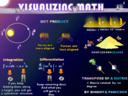# VISUALIZING MATH 2

\$4BINNOY
2 ratings

### Visualizing Math 2 deals with concepts like

1) How Fourier Transforms are the side-view of a wave.

2) Laplace Transforms are side-view + front view (Imaginary + real part)

3) How Z Transforms are nothing but the discrete cousin of Laplace and DFT of Fourier.

4) How the number 1 appears actually as a fraction 1/1 in Math which means full of full.

Thus sin(90) = 1/1 = full on influence at 90 deg

Thus Max probability = 1/1 = full of full sample space

Thus cos(0) = 1/1 = full on full influence at 0 deg (when parallel)

Unit Circle radius = 1/1 = magnitude doesn't decrease/increase

throughout the rotation. Else it would                                               have been a ratio like 2/1 or 1/4 etc.

5) How Continous functions can be visualized as those functions which you can draw on a paper without lifting up the pencil.

6) How a function differentiable at a point means if you stand at that point,...

(1) You will experience a slope. Slope will exist.

(2) This slope however will not be infinite. (fully vertical)

(3) The slope will be either towards the left or the right but                    not both the directions.

(4) The slope will not wriggle like a snake (oscillate ) at that                  point.

7) How a hermitian matrix can be visualized as a any object which which exhitibits vertical symmetry. So even if it is rotated upside down about the central axis( diagonal of the matrix) you wont be able to tell the difference.

8) How probability can be visualized much more intuitively by multiplying it by 100 and thus converting it into a percentage.

Eg:- a probability of 0.2 can be converted to 0.2 x 100 = 20%

9) How probability density can be understood using an example of the Probability of finding a man after entering New-york v/s finding a  man after entering a sparsely populated desert.

And so on.........

The book is still in works and subsequent updated will be sent to your mail id for free.

Binnoy

visualizingmathsandphysics.blogspot.in

### A SEQUEL TO VISUALIZING MATH 1

The Book Visualizing Math 2 in word and pdf format is a sequel to Visualizing Math which is once again available on gumroad the link as

VISUALIZING MATH 2 WORD AND PDF FORMAT

5.0
(2 ratings)
5 stars
100%
4 stars
0%
3 stars
0%
2 stars
0%
1 star
0%
\$4

2 ratings